# 如何构建多体动力学模块中的齿轮几何

2016年 8月 17日

### 内置齿轮零件的优势“零件库”中齿轮零件分为三类：外部啮合齿轮，内部啮合齿轮与齿条。如您希望了解“零件库”中有关齿轮零件的更多信息，请阅读齿轮模拟系列的博客文章

### 基于单个齿轮构建齿轮系

“零件库”中的齿轮基本上都是单个齿轮或齿条，但在真实的应用条件下，齿轮一般都是成对使用。为此，我们要用单个的齿轮零件来构建齿轮系。为了清晰地解释构建步骤，我们将使用以下二维直齿轮副作为示例。已知的物理量如下：

• 第一个齿轮的位置（x_1,y_1
• 第一个齿轮的节圆半径（r_1
• 第二个齿轮的节圆半径（r_2
• 第二个齿轮的角位置（\theta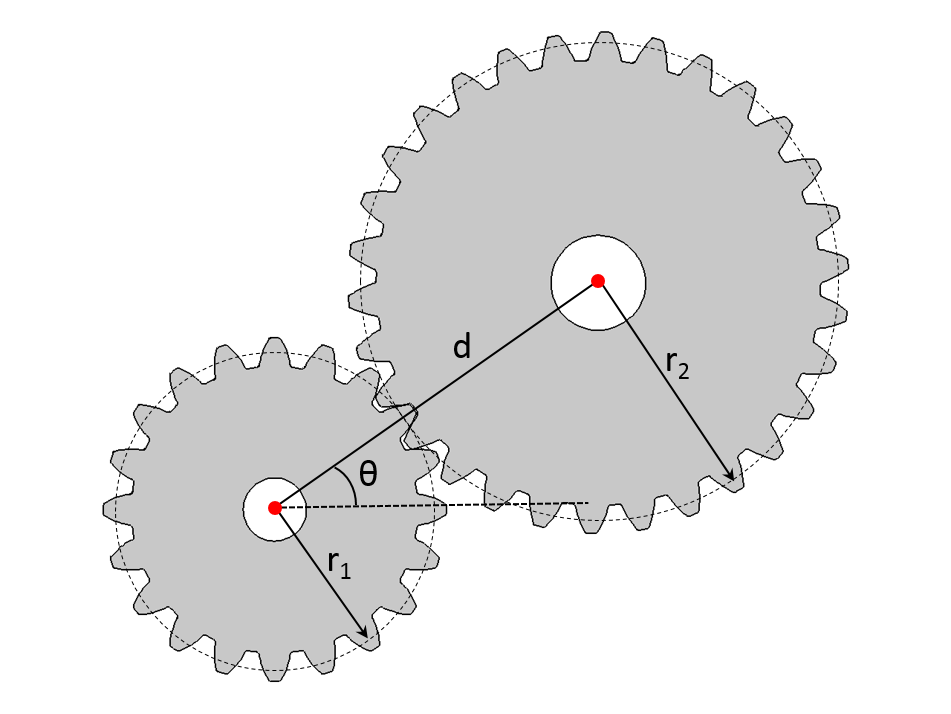d = r_1+r_2

x_2 = x_1 +d \cos{\theta}
y_2 = y_1 +d \sin{\theta}

\theta_a = \frac{\pi}{n_2}+\mod \left(\frac{\theta}{\theta_{m1}}\right)\frac{n_1}{n_2}+\mod \left(\frac{\theta}{\theta_{m2}}\right)

\theta_{m1}=\frac{2\pi}{n1};\,\,\,\,\,\,\,\,\,\,\,\,\,\,\theta_{m2}=\frac{2\pi}{n2}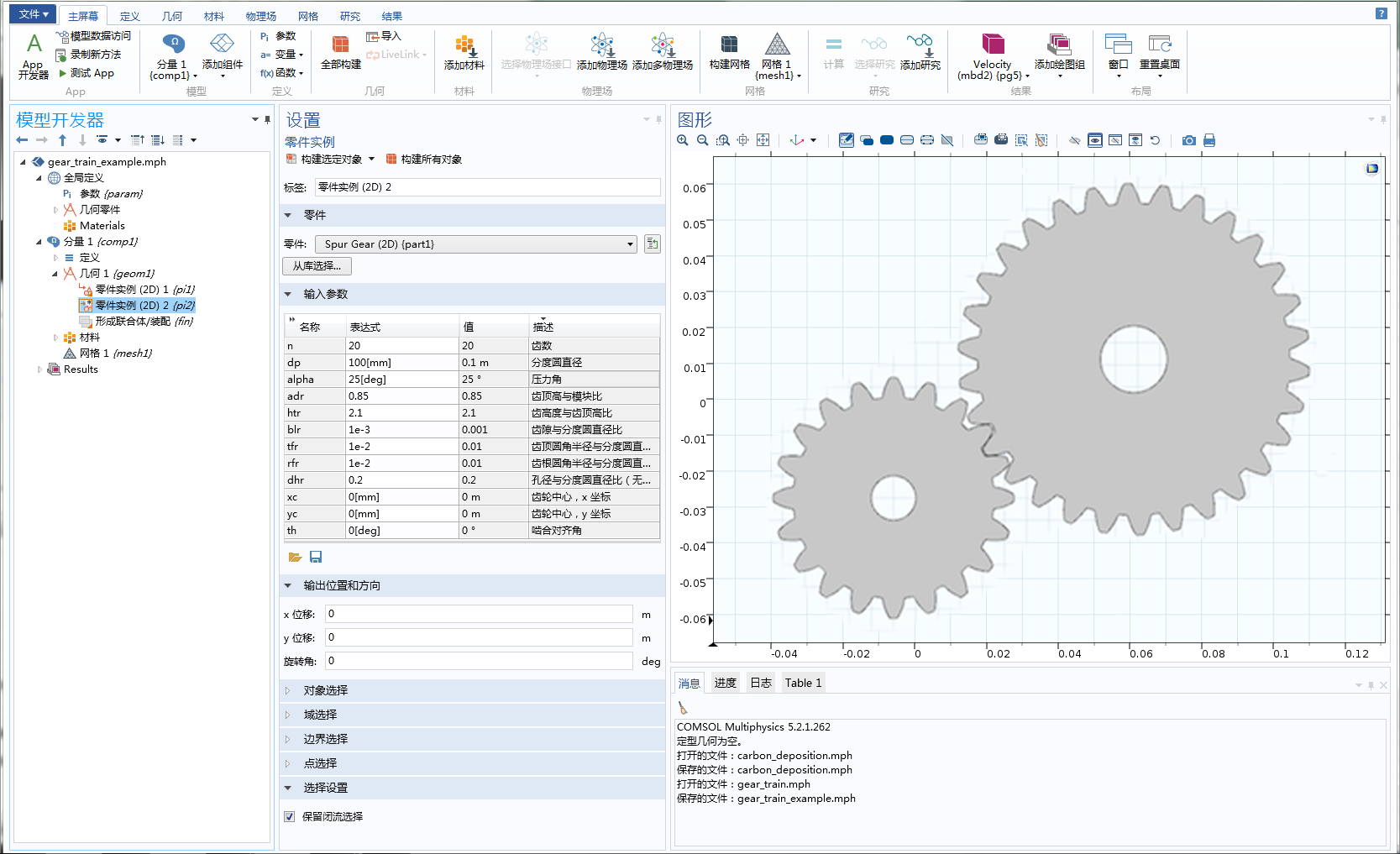### 设立齿轮参数

• 齿数（n
• 分度圆直径（dp
• 压力角（\alpha
• 螺旋角（\beta
• 齿高度与齿顶高比（htr
• 齿隙与分度圆直径比（blr
• 齿顶圆角半径与分度圆直径比（tfr
• 齿根圆角半径与分度圆直径比（rfr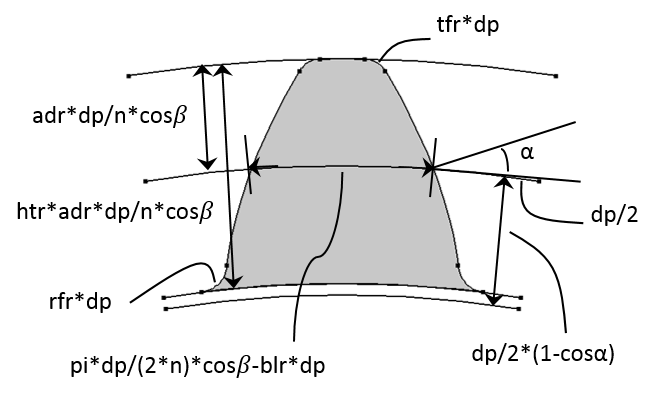• 法向模数：m = dp/n*cos\beta
• 齿高度：ht = htr*m
• 基圆直径：db = dp*\cos\alpha
• 齿顶圆角半径：tf = tfr*dp
• 齿根圆角半径：rf = rfr*dp
• 节圆处的齿厚度：t = \pi *m/2 -blr*dp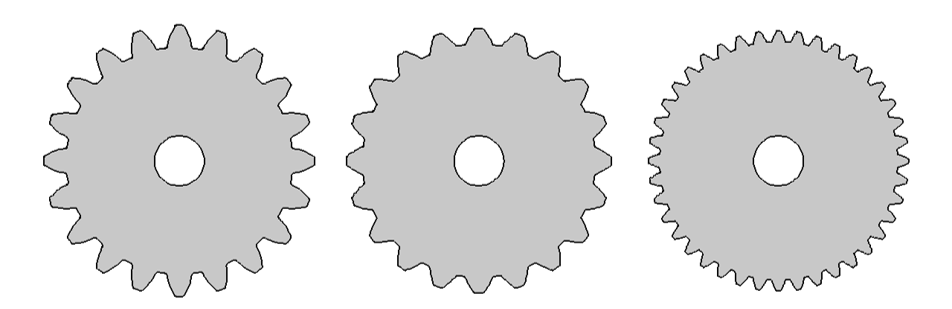### 齿轮毛坯和轴的几何模型

• 齿轮宽度与分度圆直径比（wgr
• 环宽度与齿轮宽度比（wrr
• 环外径与齿根直径比（dorr
• 环内径与孔径比（dirr

• 轴长与分度圆直径比（lsr
• 轴中心的相对轴向位置（zs

• 齿轮中心（\{xc, yc, zc\}
• 齿轮轴（\{egx, egy, egz\}

• 啮合对齐角（th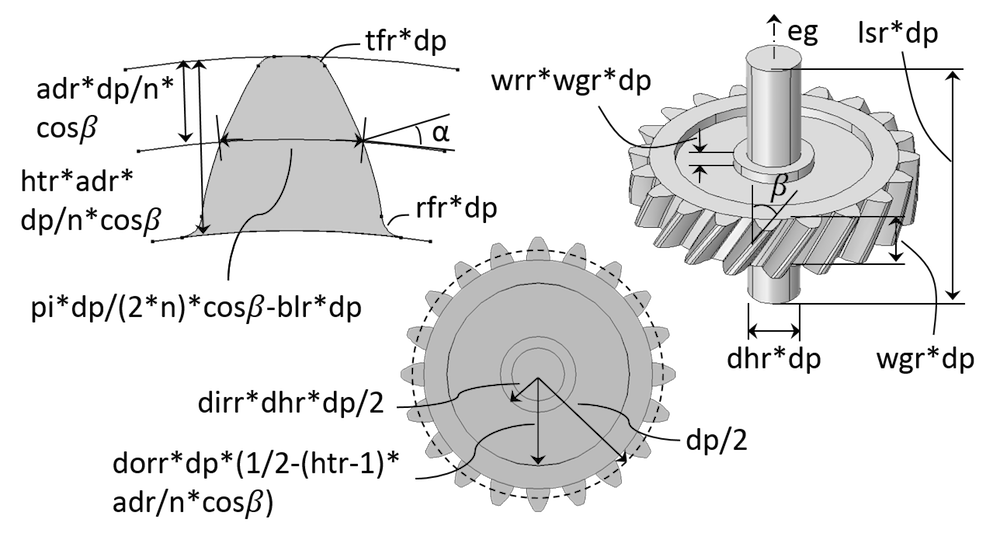• 齿轮宽度：wg = wgr*dp
• 齿环宽度：wr = wrr*wg
• 齿环外径：dor = dorr*dr
• 齿环内径：dir = dirr*dh
• 轴长：ls = lsr*dp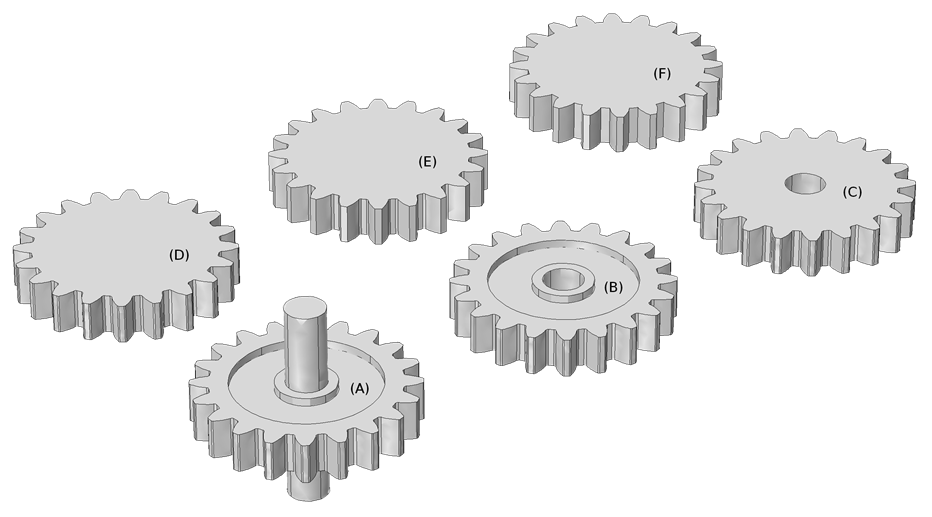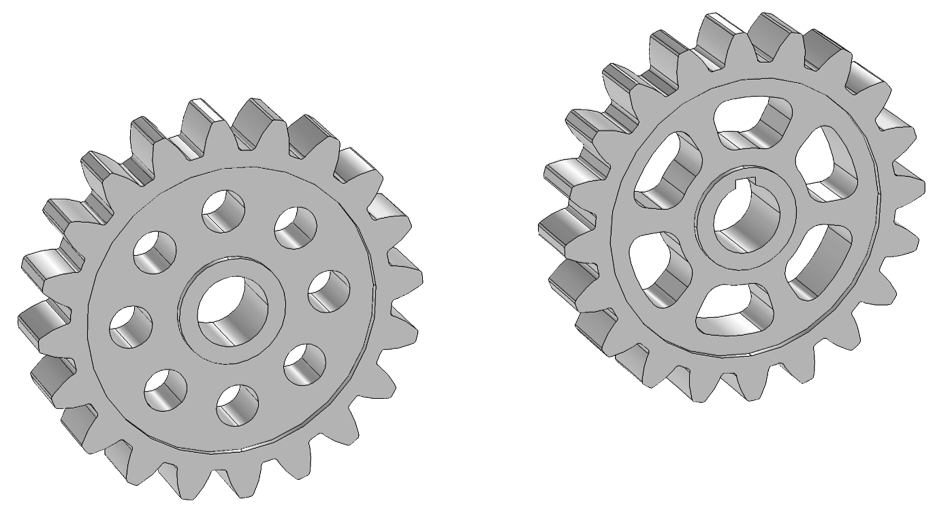### 齿轮零件库中的选择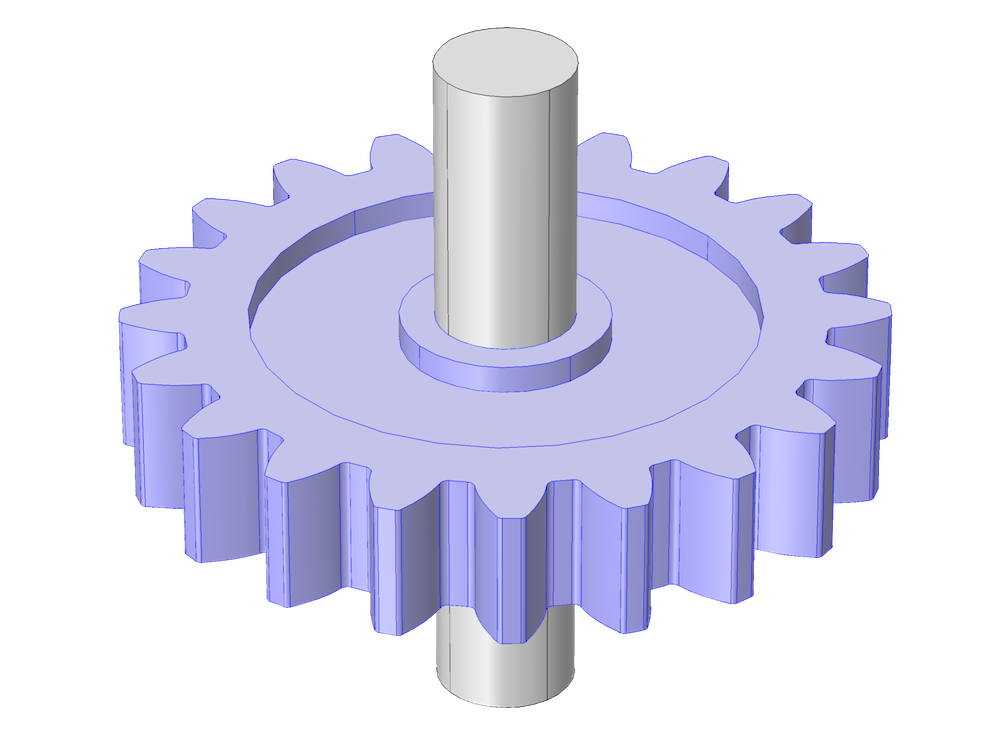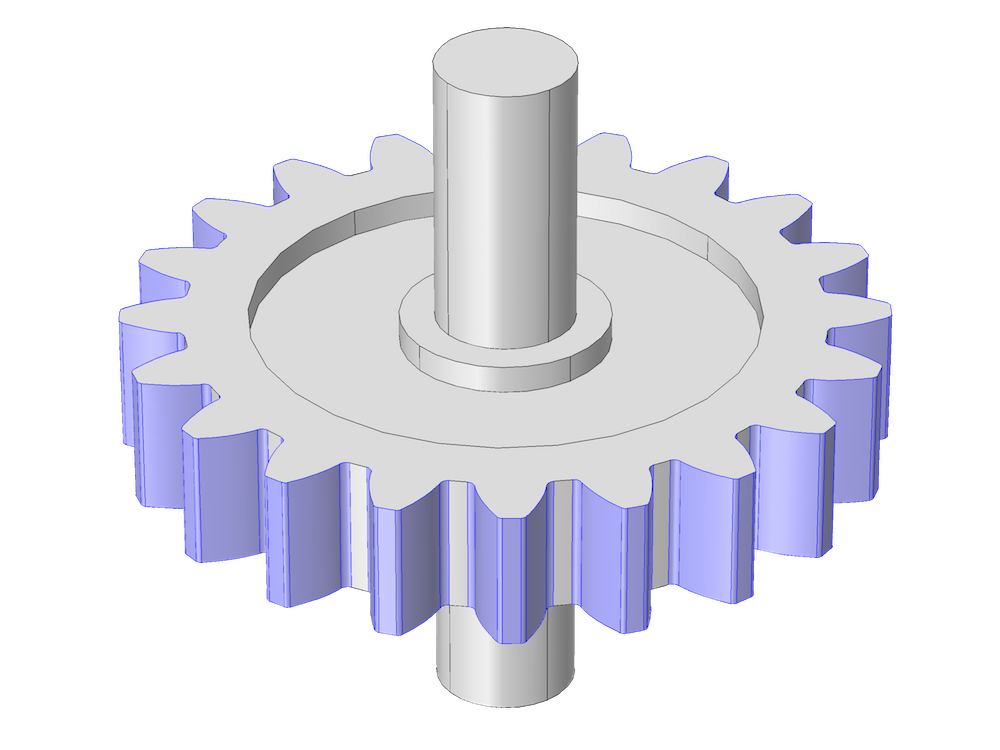### 检验及验证输入数据

• 齿根检查：(2*dd/dp)<=0.9
• 孔径检查：dh<(dp-2*dd)

### 应用案例：差动轮系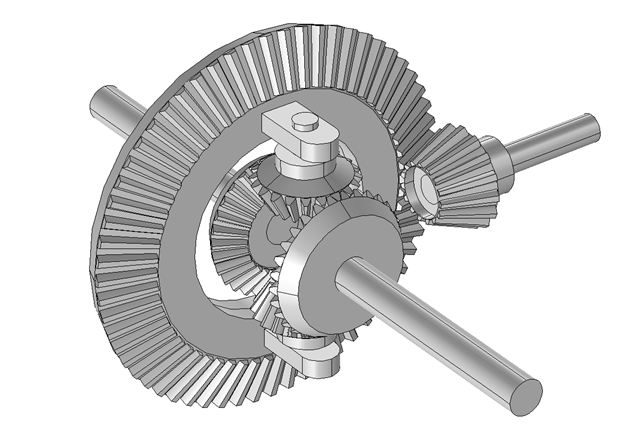### 应用案例：三级风机齿轮箱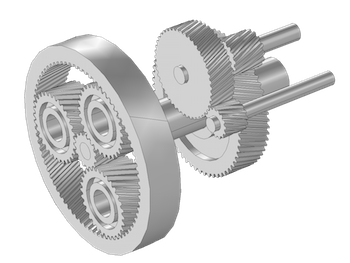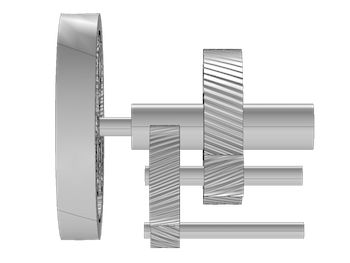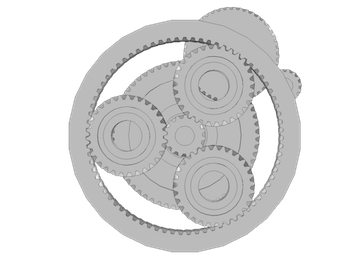### 学习更多关于齿轮建模和多体动力学模块的知识

#### 评论 (1)

##### 留言2023-04-15